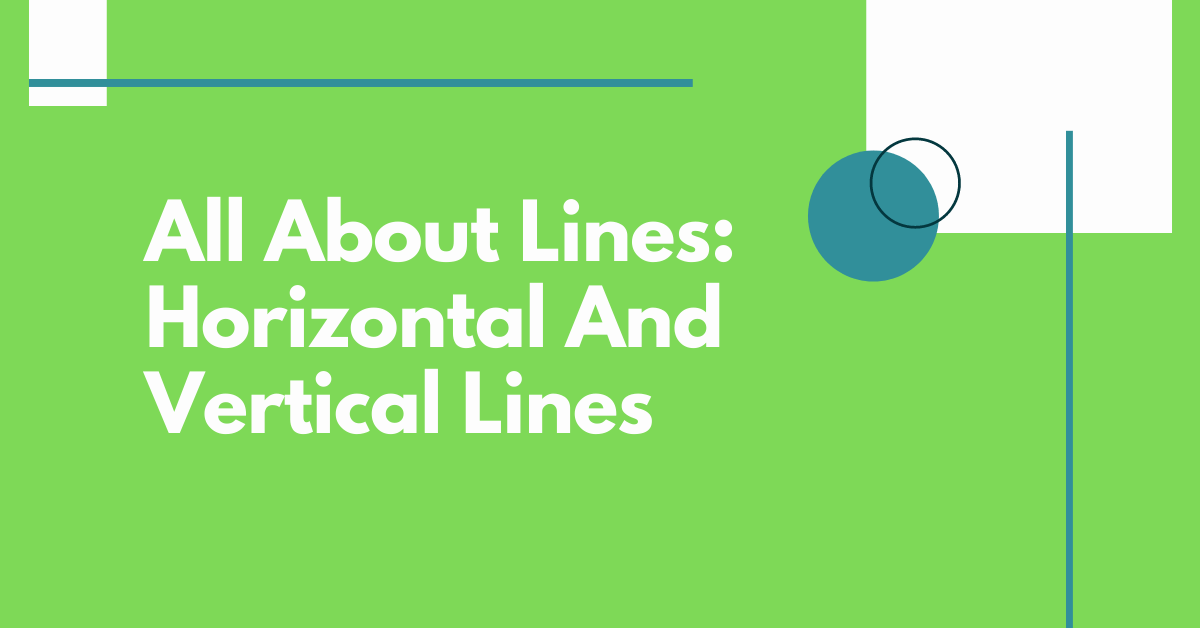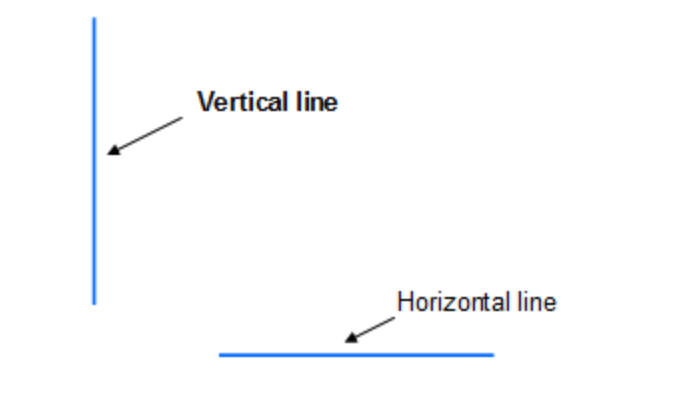You are here
Home > Features > All About Lines: Horizontal And Vertical Lines

# All About Lines: Horizontal And Vertical LinesA horizontal line is a straight line that runs from left to right or right to left in the coordinate plane and is parallel to the x-axis. In other terms, a horizontal line is a straight line with an intercept solely on the y-axis and not on the x-axis. A horizontal line serves as the foundation for flat forms. Let’s look at the qualities of the horizontal line, as well as its equation and slope.

The term “sleeping lines” refers to horizontal lines. Horizontal lines are those that are parallel to the x-axis in coordinate geometry. Horizontal line segments may be found in various shapes in geometry, including quadrilaterals, 3D shapes, and so on. Horizontal lines can be found on the steps of a staircase, boards on railway tracks, and other places in real life.

## Horizontal Line SlopeThe slope of a horizontal line is either undefined or infinite. Note that the definition of “slope” in the coordinate plane involves finding rise over run between two points on the line, but there are no points that correspond uniquely to any other point on the line. So, while the equation may be valid, it will not have an answer for its slope because there are no values for rise and run.

A horizontal line has a slope of zero. We can observe that there is no increase in the y-coordinates since they are the same across the horizontal line when we calculate slope = rise/run. As a result, the y coordinates do not change, and the horizontal line’s slope finally becomes 0.

## Equation of a Horizontal Line

All of the points on a horizontal line have the same y-coordinates. Thus, a horizontal line passing through any point (a,b) has the equation y = b, where b is constant.

x isn’t there in this case. It indicates that the x-coordinate of any point on the line can be anything, but the y-coordinate of all points on the line must be just ‘b’. The horizontal line’s y-intercept is (0, b)

We can observe that the y-coordinate of all these places is constant, which is -3, if we consider the preceding picture points (a,b): (2, -3), (1, -3), (-2, -3). As a result, this line’s equation is y = -3.

## Vertical LineA vertical line is a line in the coordinate plane with the same x-coordinate for all of its points. When charting the points for a function x = a on a coordinate plane, we will see that connecting the coordinates yields a vertical line. If L1 and L2 are the vertical lines . For all the values of y, all the points in the L1 have only ‘a’ as the x-coordinate, and all the points in the L2 have only ‘-a’ as the x-coordinate.

A Vertical Line Equation is a formula for calculating the length of a vertical line.

x = a or x = -a is the equation for a vertical line, where

The x-coordinate of any point on a line is x.

The point at which the line crosses the x-intercept is called a.

## Vertical Line Properties

Because the line is parallel to the y-axis, the vertical line’s equation does not have a y-intercept .A vertical line’s equation is always of the form x = a, where ‘a’ represents the x-intercept. A vertical line’s slope is unspecified. The denominator of the slope is 0 since the x-coordinates do not change.

In arithmetic, the vertical line is used to determine if a relationship is a function. The vertical line L1 crosses the x-axis at (0, 0) and L2 crosses the x-axis at (-a, 0). The vertical line’s equation is written as y = b or in slope intercept form is y – b = 0. The point of intersection with the x axis occurs when y – b = 0 which is equivalent to 2x + 3y – 6 = 0. This equation simplifies to x + y – 3 = 0 for L1 or x – y + 3 = for L2.

This was some basic information on lines and one can explore more fascinating information on the Cuemath website.

INTERESTING POSTS

This site uses Akismet to reduce spam. Learn how your comment data is processed.